### Home > PC > Chapter 4 > Lesson 4.1.1 > Problem4-11

4-11.
1. Simplify each of the following expressions into a single reduced fraction. Homework Help ✎

1.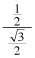2.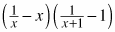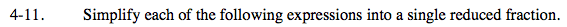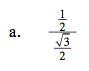Multiply the numerator by the reciprocal of the denominator.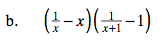$\left(\frac{1-x^2}{x}\right)\left(\frac{1-x-1}{x+1}\right)$